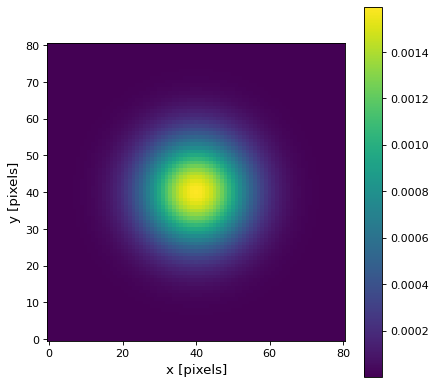# Gaussian2DKernel¶

class `astropy.convolution.``Gaussian2DKernel`(x_stddev, y_stddev=None, theta=0.0, **kwargs)[source]

2D Gaussian filter kernel.

The Gaussian filter is a filter with great smoothing properties. It is isotropic and does not produce artifacts.

Parameters
x_stddev`float`

Standard deviation of the Gaussian in x before rotating by theta.

y_stddev`float`

Standard deviation of the Gaussian in y before rotating by theta.

theta

Rotation angle. If passed as a float, it is assumed to be in radians. The rotation angle increases counterclockwise.

x_size`int`, optional

Size in x direction of the kernel array. Default = ⌊8*stddev + 1⌋.

y_size`int`, optional

Size in y direction of the kernel array. Default = ⌊8*stddev + 1⌋.

mode`str`, optional
One of the following discretization modes:
• ‘center’ (default)

Discretize model by taking the value at the center of the bin.

• ‘linear_interp’

Discretize model by performing a bilinear interpolation between the values at the corners of the bin.

• ‘oversample’

Discretize model by taking the average on an oversampled grid.

• ‘integrate’

Discretize model by integrating the model over the bin.

factornumber, optional

Factor of oversampling. Default factor = 10.

Examples

Kernel response:

```import matplotlib.pyplot as plt
from astropy.convolution import Gaussian2DKernel
gaussian_2D_kernel = Gaussian2DKernel(10)
plt.imshow(gaussian_2D_kernel, interpolation='none', origin='lower')
plt.xlabel('x [pixels]')
plt.ylabel('y [pixels]')
plt.colorbar()
plt.show()
```

(png, svg, pdf)